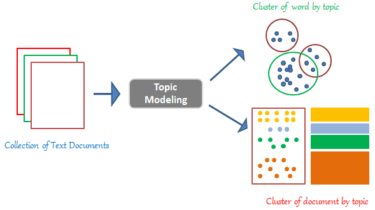# Topic Modelling With LDA -A Hands-on IntroductionThis article was published as a part of the Data Science Blogathon

## Introduction

Imagine walking into a bookstore to buy a book on world economics and not being able to figure out the section of the store that has this book, assuming the bookstore has simply stacked all types of books together. You then realize how important it is to divide the bookstore into different sections based on the type of book.

Topic Modelling is similar to dividing a bookstore based on the content of the books as it refers to the process of discovering themes in a text corpus and annotating the documents based on the identified topics.

When you need to segment, understand, and summarize a large collection of documents, topic modelling can be useful.

## Topic Modelling using LDA:

Latent Dirichlet Allocation (LDA) is one of the ways to implement Topic Modelling. It is a generative probabilistic model in which each document is assumed to be consisting of a different proportion of topics.